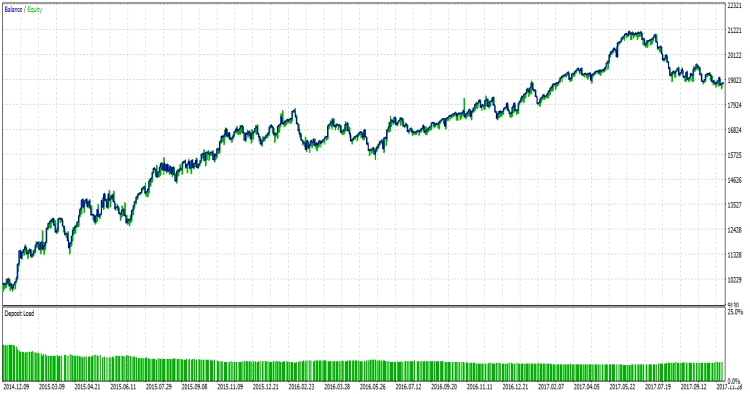# Larry Conners RSI 2 – expert for MetaTrader 5

This Expert Advisor uses three indicators, RSI (Relative Strength Index), fast Moving Average (5 period), slow Moving Average (200 period).

The Idea of this strategy is to determine the general trend by using the slow Moving Average (MA 200). Then buy on the dips and sell on the rises.

Long Entry Rules

• RSI (2 period) is less than 6.
• Close of previous candle is greater than slow Moving Average.

Long Exit Rules

• Close is greater than fast Moving Average.

Short Entry Rules

• RSI (2 period) is greater than 95.
• Close of previous candle is less than slow Moving Average.
Related Posts

Short Exit Rules

• Close is less than fast Moving Average.

Input parameters

```input double   lot = 1;                  //Lots
input int      shortSmaPeriods = 5;      //Fast MA period
input int      longSmaPeriods = 200;     //Slow MA period
input int      RSIPeriods = 2;           //RSI Period
input int      RSILongEntry = 6;         //RSI Long Entry
input int      RSIShortEntry = 95;       //RSI Short Entry

input int      slippage=3;
input bool     useStopLoss=true;       //Use Stop Loss
input double   stopLossPips=30;        //Stop Loss (pips)
input bool     useTakeProfit=true;     //Use Take Profit
input double   takeProfitPips=60;      //Take Profit (pips)```

Best results on the EUR/USD pair 1H timeframe. Play with inputs to find your desired results.